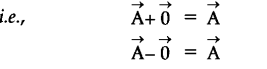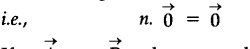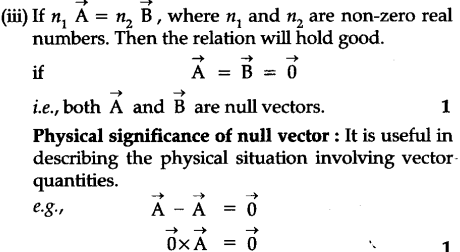# Define null vector. What are its properties ? What is its physical significance?

It is defined as a vector having zero magnitude and acting in the arbitrary direction. It is denoted by 0.

Properties of null vector:
(i) The addition or subtraction of zero vector from a given vector is again the same vector(ii) The multiplication of zero vector by a non-zero real number is again the zero vector.